Next: Supersonic Expansion by Turning Up: Two-Dimensional Compressible Inviscid Flow Previous: Weak Oblique Shocks

# Supersonic Compression by Turning

A shock front increases the pressure and density of the fluid that passes through it. In other words, the front compresses the flow. A simple method for compressing a supersonic flow is to turn it through an oblique shock, by deflecting the wall through an angle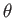, as shown in Figure 15.6(a).

The wall deflection can be subdivided into several segments, which make smaller corners of angle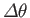, as shown in Figure 15.6(b). Compression then occurs through successive oblique shocks. These shocks divide the flow field close to the wall into segments of uniform flow. Further out, the shocks must intersect one another, because they are convergent. However, for the present, we shall only consider the flow close to the wall. In this region, each segment of the flow is independent of the following segment. In other words, the flow pattern can be constructed step by step, proceeding downstream. This property of limited upstream influence exists as long as the deflection does not become so large that the flow is rendered subsonic.

To compare the compression in the two cases, (a) and (b), of Figure 15.6, we shall make use of the approximate expressions for weak shocks obtained in the preceding section. For each shock in case (b), we have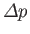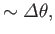(15.30)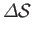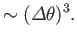(15.31)

Thus, if the complete turn is subdivided into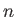equal segments, so that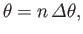(15.32)

then the overall pressure and specific entropy changes are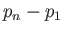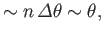(15.33)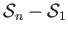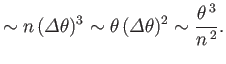(15.34)

Hence, if the compression is achieved by means of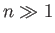weak shocks then the net specific entropy increase can be reduced very significantly, compared to that generated by a single shock that produces the same deflection. In fact, the reduction is by a factor of order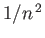.

In the limit that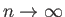, the smooth turn of Figure 15.6(c) is obtained. In this case, the net increase in specific entropy becomes vanishingly small. In other words, the compression becomes isentropic. We can also deduce the following results. First, the shocks become vanishingly weak, their limiting positions being straight Mach lines emanating from the wall. Second, each segment of uniform flow becomes vanishingly narrow, and eventually coincides with a Mach line. Thus, on each Mach line, both the flow inclination and the Mach number are constant. Third, the limited upstream influence is preserved. That is, the flow upstream of a given Mach line is not affected by any downstream modifications in the wall. Finally, the approximate expression for the change in flow speed across a weak shock, Equation (15.29), becomes the differential expression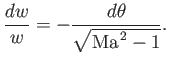(15.35)

Let us now consider what happens to the flow further away from the wall, where the weak shocks and Mach lines of Figure 15.6(b) and Figure 15.6(c), respectively, converge. Consider Figure 15.7. Because of the convergence of the Mach lines, the change from the initial Mach number,, to the final Mach number,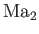, is achieved in a much shorter distance on streamline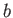than on streamline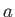. Thus, the velocity gradients on streamlineare higher than those on streamline. Now, an intersection of Mach lines would imply infinitely high velocity gradients, because there would be two different values of the Mach number at a single point. In practice, this does not occur, because, in the region where the Mach lines converge, and before they cross, the velocity gradients become high enough that the conditions are no longer isentropic. In fact, a shock wave develops, as illustrated in the figure.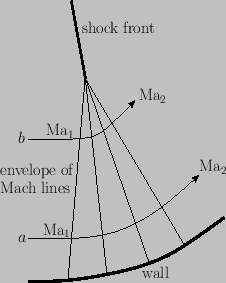Next: Supersonic Expansion by Turning Up: Two-Dimensional Compressible Inviscid Flow Previous: Weak Oblique Shocks
Richard Fitzpatrick 2016-03-31